# Arrays in Data Structure and Algorithm

21. Sep 2021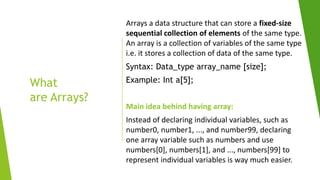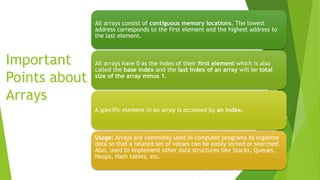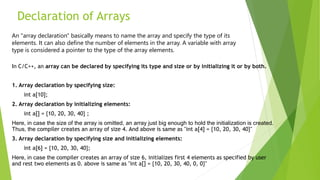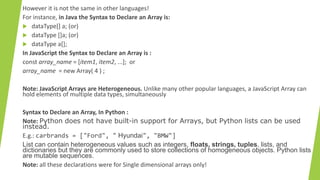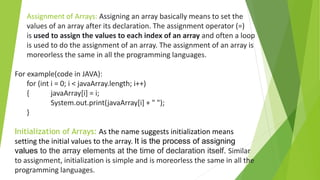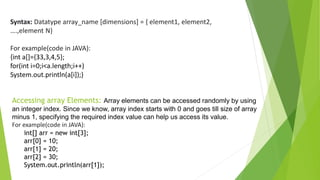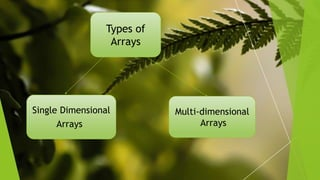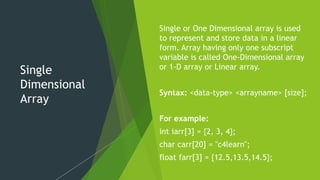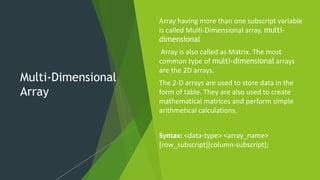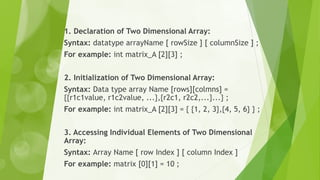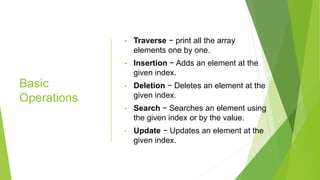1 von 12

### Arrays in Data Structure and Algorithm

• 1. Introduction to Arrays in Data Structures and Algorithm By Kristina Barooah Computer Science Engineering, Lovely Professional University Technical Member of GeeksforGeeks-LPU.
• 2. What are Arrays? Arrays a data structure that can store a fixed-size sequential collection of elements of the same type. An array is a collection of variables of the same type i.e. it stores a collection of data of the same type. Syntax: Data_type array_name [size]; Example: Int a; Main idea behind having array: Instead of declaring individual variables, such as number0, number1, ..., and number99, declaring one array variable such as numbers and use numbers, numbers, and ..., numbers to represent individual variables is way much easier.
• 3. Important Points about Arrays All arrays consist of contiguous memory locations. The lowest address corresponds to the first element and the highest address to the last element. All arrays have 0 as the index of their first element which is also called the base index and the last index of an array will be total size of the array minus 1. A specific element in an array is accessed by an index. Usage: Arrays are commonly used in computer programs to organize data so that a related set of values can be easily sorted or searched. Also, used to Implement other data structures like Stacks, Queues, Heaps, Hash tables, etc.
• 4. Declaration of Arrays In C/C++, an array can be declared by specifying its type and size or by initializing it or by both. 1. Array declaration by specifying size: int a; 2. Array declaration by initializing elements: int a[] = {10, 20, 30, 40} ; Here, in case the size of the array is omitted, an array just big enough to hold the initialization is created. Thus, the compiler creates an array of size 4. And above is same as "int a = {10, 20, 30, 40}" 3. Array declaration by specifying size and initializing elements: int a = {10, 20, 30, 40}; Here, in case the compiler creates an array of size 6, initializes first 4 elements as specified by user and rest two elements as 0. above is same as "int a[] = {10, 20, 30, 40, 0, 0}" An "array declaration" basically means to name the array and specify the type of its elements. It can also define the number of elements in the array. A variable with array type is considered a pointer to the type of the array elements.
• 5. However it is not the same in other languages! For instance, in Java the Syntax to Declare an Array is:  dataType[] a; (or)  dataType []a; (or)  dataType a[]; In JavaScript the Syntax to Declare an Array is : const array_name = [item1, item2, ...]; or array_name = new Array( 4 ) ; Note: JavaScript Arrays are Heterogeneous. Unlike many other popular languages, a JavaScript Array can hold elements of multiple data types, simultaneously Syntax to Declare an Array, In Python : Note: Python does not have built-in support for Arrays, but Python lists can be used instead. E.g.: carbrands = ["Ford", " Hyundai", "BMW"] List can contain heterogeneous values such as integers, floats, strings, tuples, lists, and dictionaries but they are commonly used to store collections of homogeneous objects. Python lists are mutable sequences. Note: all these declarations were for Single dimensional arrays only!
• 6. Assignment of Arrays: Assigning an array basically means to set the values of an array after its declaration. The assignment operator (=) is used to assign the values to each index of an array and often a loop is used to do the assignment of an array. The assignment of an array is moreorless the same in all the programming languages. For example(code in JAVA): for (int i = 0; i < javaArray.length; i++) { javaArray[i] = i; System.out.print(javaArray[i] + " "); } Initialization of Arrays: As the name suggests initialization means setting the initial values to the array. It is the process of assigning values to the array elements at the time of declaration itself. Similar to assignment, initialization is simple and is moreorless the same in all the programming languages.
• 7. Syntax: Datatype array_name [dimensions] = { element1, element2, ….,element N} For example(code in JAVA): {int a[]={33,3,4,5}; for(int i=0;i<a.length;i++) System.out.println(a[i]);} Accessing array Elements: Array elements can be accessed randomly by using an integer index. Since we know, array index starts with 0 and goes till size of array minus 1, specifying the required index value can help us access its value. For example(code in JAVA): int[] arr = new int; arr = 10; arr = 20; arr = 30; System.out.println(arr);
• 8. Types of Arrays Single Dimensional Arrays Multi-dimensional Arrays
• 9. Single Dimensional Array Single or One Dimensional array is used to represent and store data in a linear form. Array having only one subscript variable is called One-Dimensional array or 1-D array or Linear array. Syntax: <data-type> <arrayname> [size]; For example: int iarr = {2, 3, 4}; char carr = "c4learn"; float farr = {12.5,13.5,14.5};
• 10. Multi-Dimensional Array Array having more than one subscript variable is called Multi-Dimensional array. multi- dimensional Array is also called as Matrix. The most common type of multi-dimensional arrays are the 2D arrays. The 2-D arrays are used to store data in the form of table. They are also used to create mathematical matrices and perform simple arithmetical calculations. Syntax: <data-type> <array_name> [row_subscript][column-subscript];
• 11. 1. Declaration of Two Dimensional Array: Syntax: datatype arrayName [ rowSize ] [ columnSize ] ; For example: int matrix_A  ; 2. Initialization of Two Dimensional Array: Syntax: Data type array Name [rows][colmns] = {{r1c1value, r1c2value, ...},{r2c1, r2c2,...}...} ; For example: int matrix_A  = { {1, 2, 3},{4, 5, 6} } ; 3. Accessing Individual Elements of Two Dimensional Array: Syntax: Array Name [ row Index ] [ column Index ] For example: matrix  = 10 ;
• 12. Basic Operations • Traverse − print all the array elements one by one. • Insertion − Adds an element at the given index. • Deletion − Deletes an element at the given index. • Search − Searches an element using the given index or by the value. • Update − Updates an element at the given index.